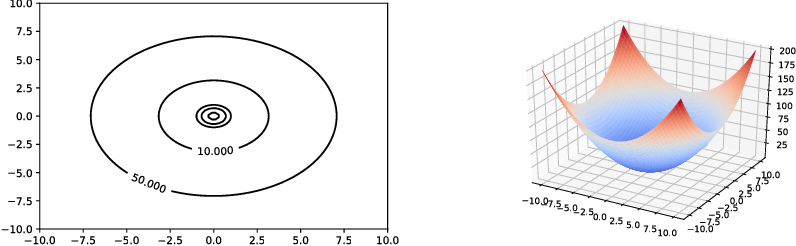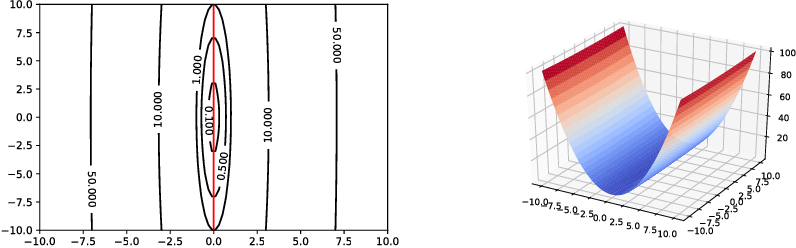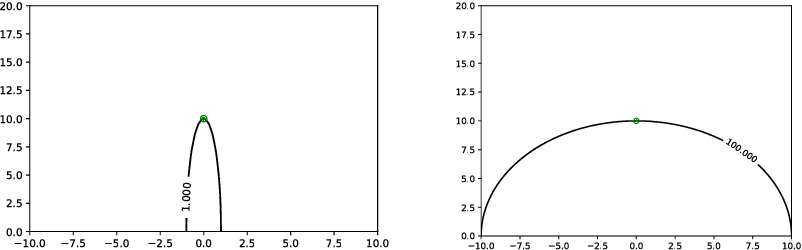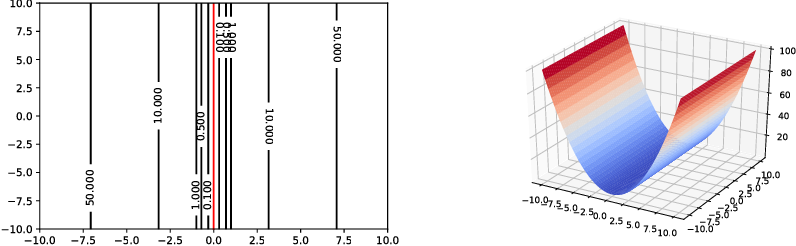The word "valley" is a popular term used in intuitively describing fitness landscapes. What is a valley on a fitness landscape? How to identify the direction and location of a valley if it exists? However, such questions are seldom rigorously studied in evolutionary optimization especially when the search space is a high dimensional continuous space. This paper presents two methods of studying valleys on a fitness landscape. The first method is based on the topological homeomorphism. It establishes a rigorous definition of a valley. A valley is regarded as a one-dimensional manifold. The second method takes a different viewpoint from statistics. It provides an algorithm of identifying the valley direction and location using principle component analysis.

Click to Read Paper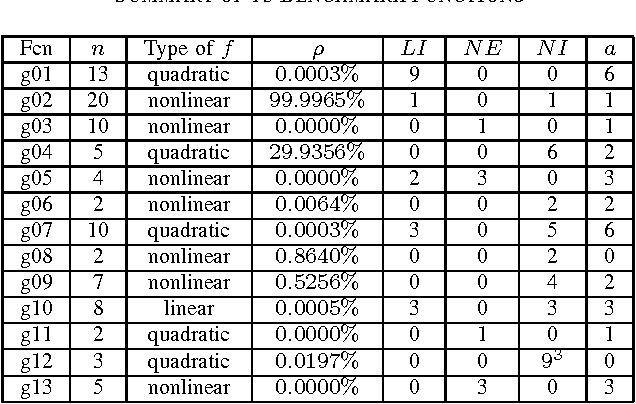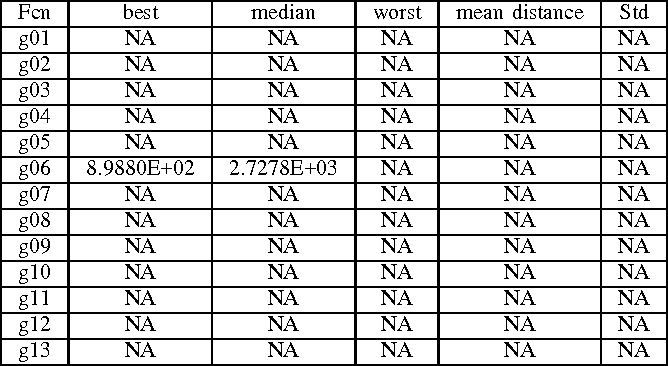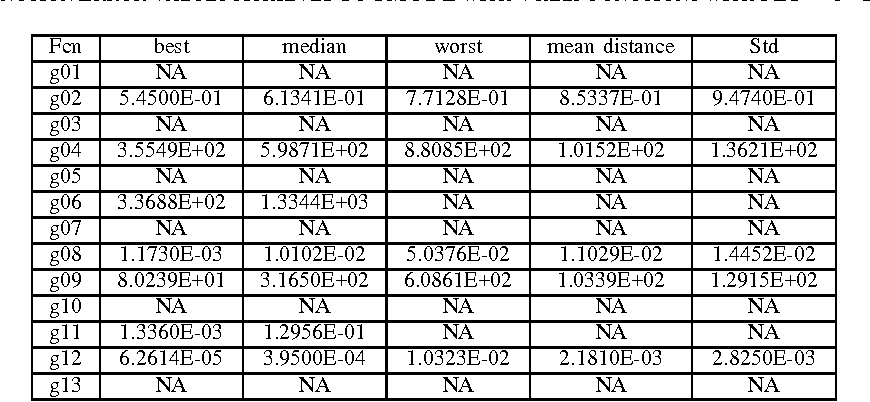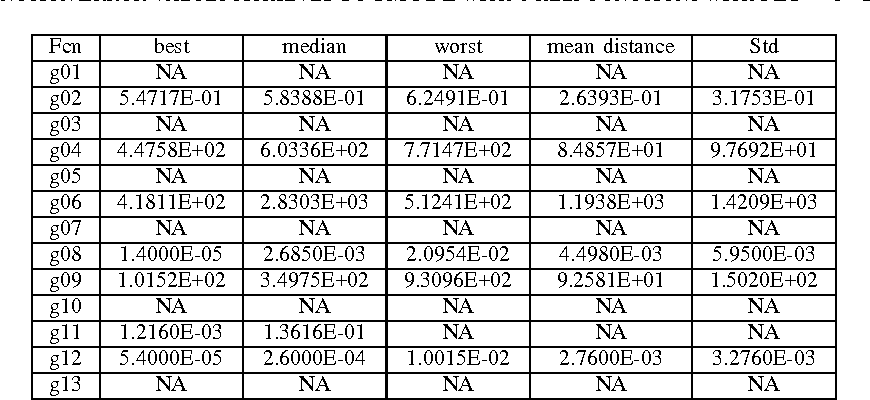Solving constrained optimization problems by multi-objective evolutionary algorithms has scored tremendous achievements in the last decade. Standard multi-objective schemes usually aim at minimizing the objective function and also the degree of constraint violation simultaneously. This paper proposes a new multi-objective method for solving constrained optimization problems. The new method keeps two standard objectives: the original objective function and the sum of degrees of constraint violation. But besides them, four more objectives are added. One is based on the feasible rule. The other three come from the penalty functions. This paper conducts an initial experimental study on thirteen benchmark functions. A simplified version of CMODE is applied to solving multi-objective optimization problems. Our initial experimental results confirm our expectation that adding more helper functions could be useful. The performance of SMODE with more helper functions (four or six) is better than that with only two helper functions.

* Accepted by The 15th UK Workshop on Computational Intelligence (UKCI 2015)
Click to Read Paper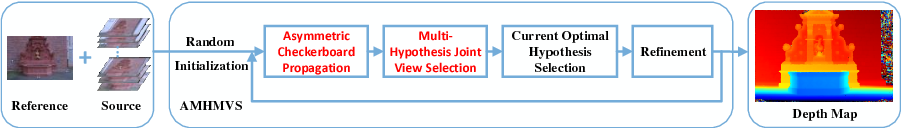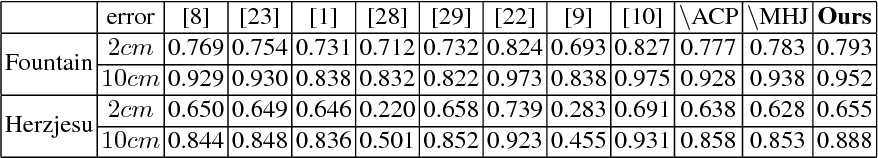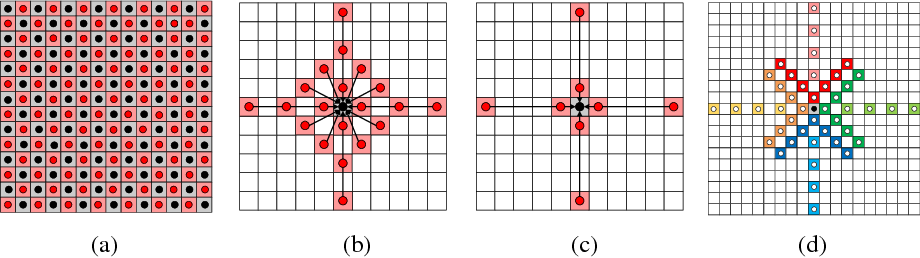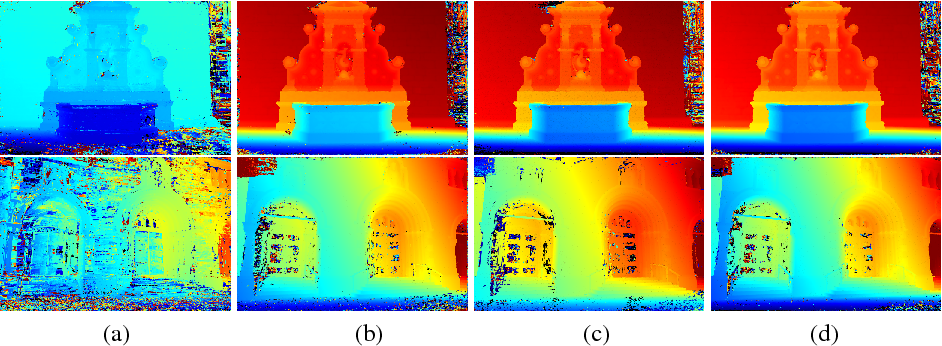In computer vision domain, how to fast and accurately perform multiview stereo (MVS) is still a challenging problem. In this paper we present a fast yet accurate method for 3D dense reconstruction, called AMHMVS, built on the PatchMatch based stereo algorithm. Different from the regular symmetric propagation scheme, our approach adopts an asymmetric checkerboard propagation strategy, which can adaptively make effective hypotheses expand further according to the confidence of current neighbor hypotheses. In order to aggregate visual information from multiple images better, we propose the multi-hypothesis joint view selection for each pixel, which leverages a cost matrix based on the multiple propagated hypotheses to robustly infer an appropriate aggregation subset parallel. Combined with the above two steps, our approach not only has the capacity of massively parallel computation, but also obtains high accuracy and completeness. Experiments on extensive datasets show that our method achieves more accurate and robust results, and runs faster than the competing methods.

Click to Read Paper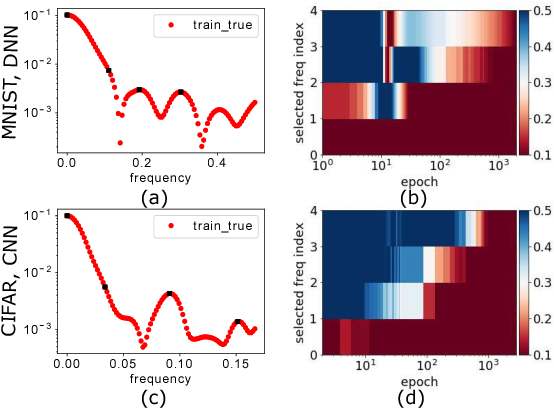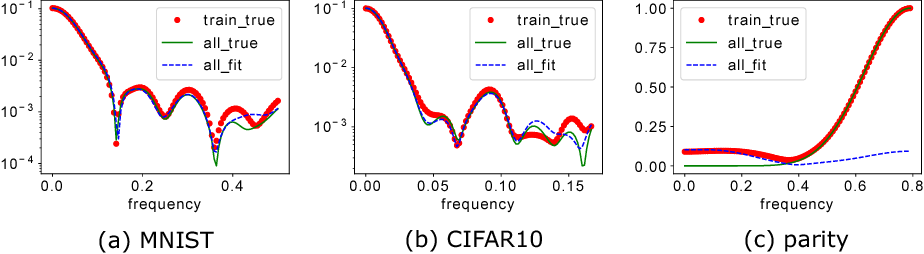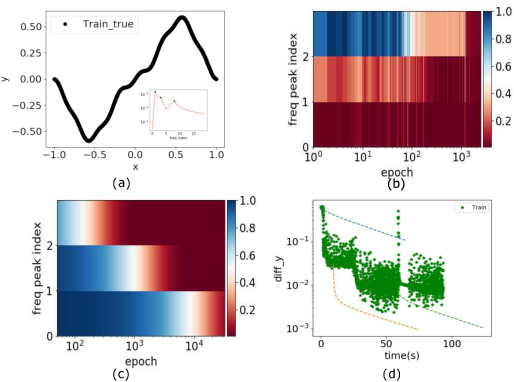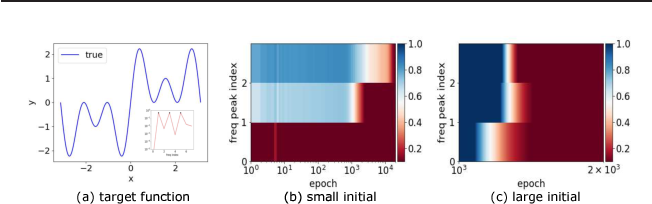We study the training process of Deep Neural Networks (DNNs) from the Fourier analysis perspective. Our starting point is a Frequency Principle (F-Principle) --- DNNs initialized with small parameters often fit target functions from low to high frequencies --- which was first proposed by Xu et al. (2018) and Rahaman et al. (2018) on synthetic datasets. In this work, we first show the universality of the F-Principle by demonstrating this phenomenon on high-dimensional benchmark datasets, such as MNIST and CIFAR10. Then, based on experiments, we show that the F-Principle provides insight into both the success and failure of DNNs in different types of problems. Based on the F-Principle, we further propose that DNN can be adopted to accelerate the convergence of low frequencies for scientific computing problems, in which most of the conventional methods (e.g., Jacobi method) exhibit the opposite convergence behavior --- faster convergence for higher frequencies. Finally, we prove a theorem for DNNs of one hidden layer as a first step towards a mathematical explanation of the F-Principle. Our work indicates that the F-Principle with Fourier analysis is a promising approach to the study of DNNs because it seems ubiquitous, applicable, and explainable.

* 7 pages, 4 figures, under review of ICML
Click to Read Paper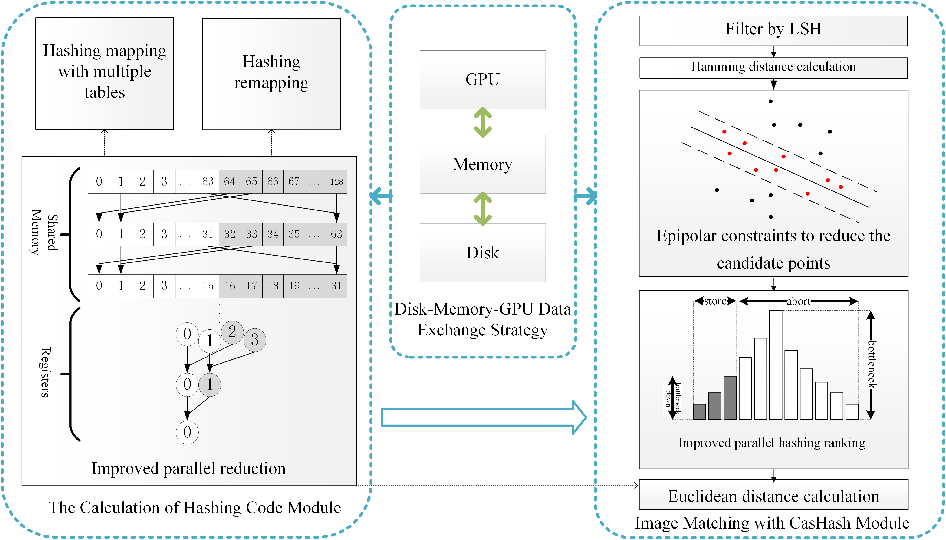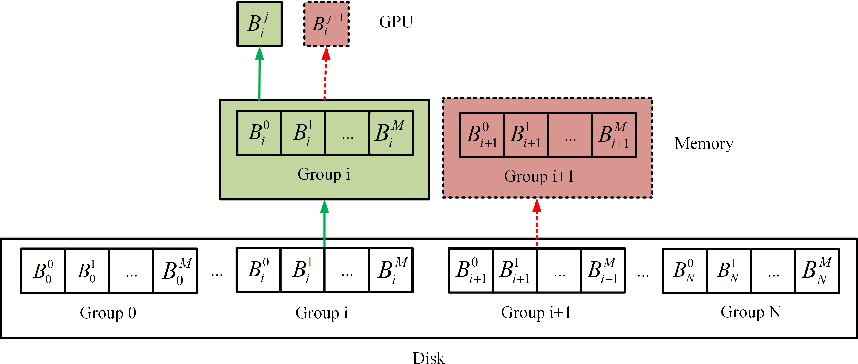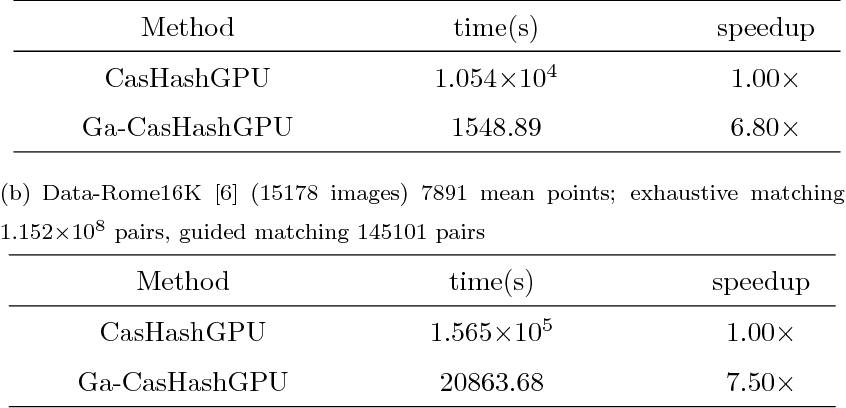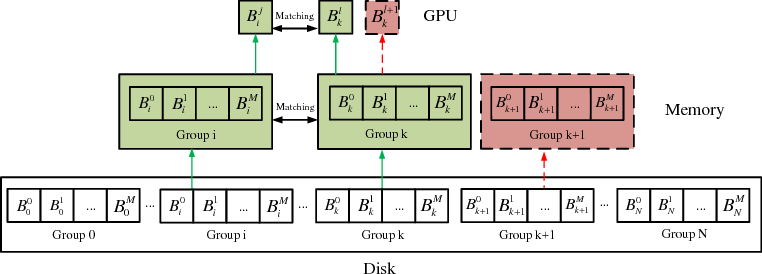Image feature point matching is a key step in Structure from Motion(SFM). However, it is becoming more and more time consuming because the number of images is getting larger and larger. In this paper, we proposed a GPU accelerated image matching method with improved Cascade Hashing. Firstly, we propose a Disk-Memory-GPU data exchange strategy and optimize the load order of data, so that the proposed method can deal with big data. Next, we parallelize the Cascade Hashing method on GPU. An improved parallel reduction and an improved parallel hashing ranking are proposed to fulfill this task. Finally, extensive experiments show that our image matching is about 20 times faster than SiftGPU on the same graphics card, nearly 100 times faster than the CPU CasHash method and hundreds of times faster than the CPU Kd-Tree based matching method. Further more, we introduce the epipolar constraint to the proposed method, and use the epipolar geometry to guide the feature matching procedure, which further reduces the matching cost.

Click to Read Paper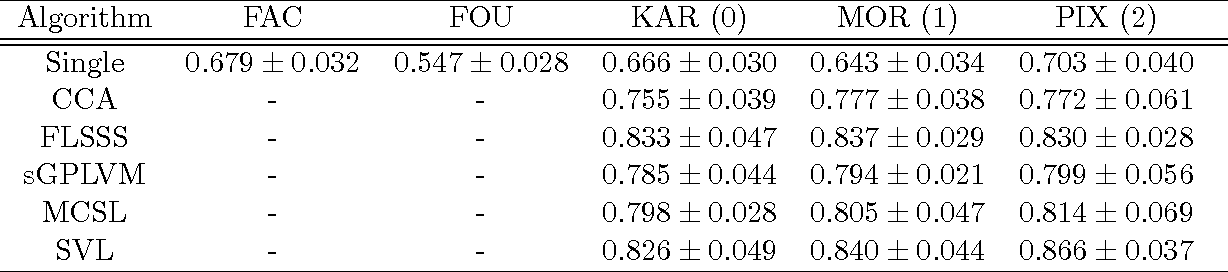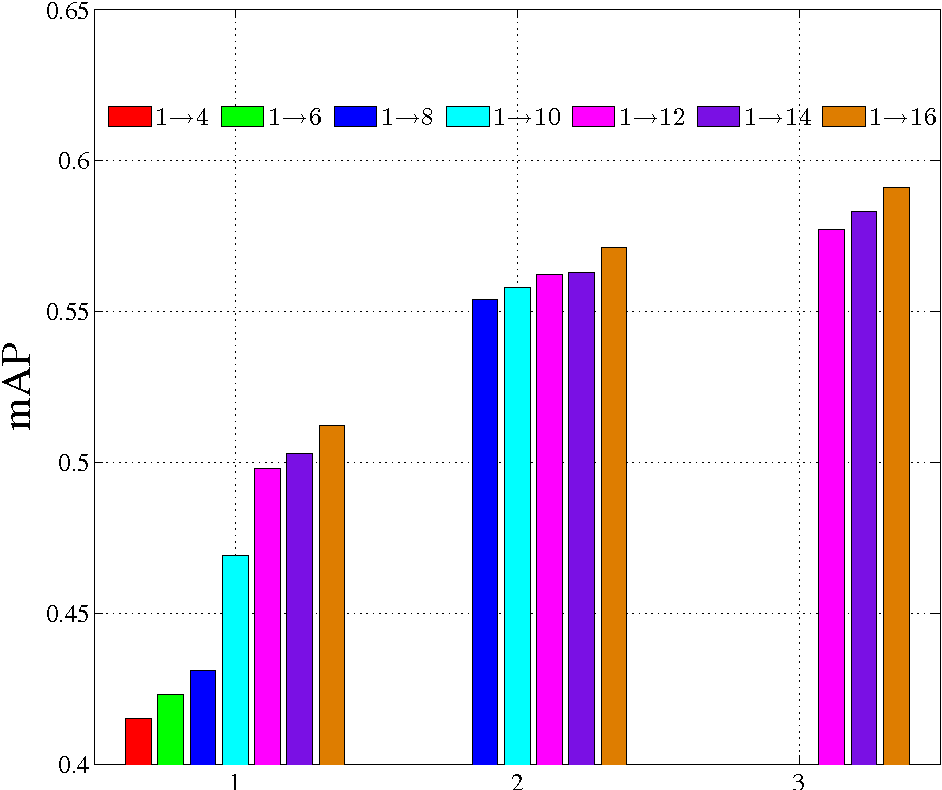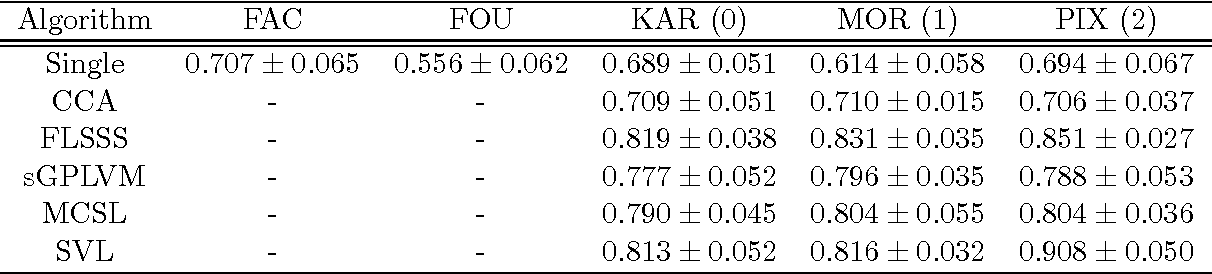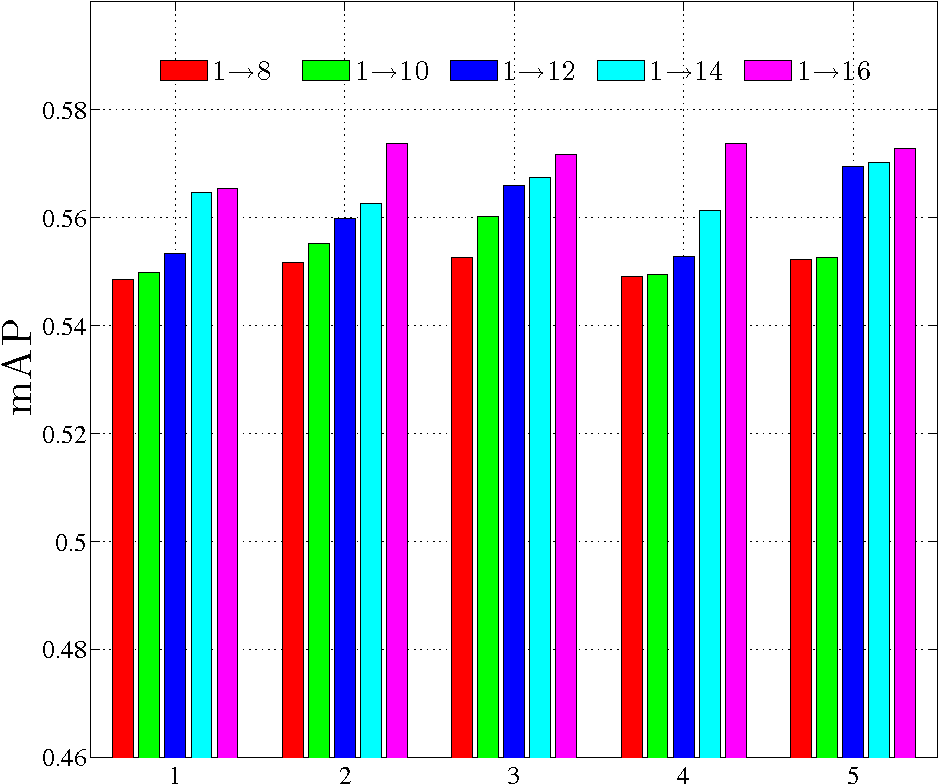An underlying assumption in conventional multi-view learning algorithms is that all views can be simultaneously accessed. However, due to various factors when collecting and pre-processing data from different views, the streaming view setting, in which views arrive in a streaming manner, is becoming more common. By assuming that the subspaces of a multi-view model trained over past views are stable, here we fine tune their combination weights such that the well-trained multi-view model is compatible with new views. This largely overcomes the burden of learning new view functions and updating past view functions. We theoretically examine convergence issues and the influence of streaming views in the proposed algorithm. Experimental results on real-world datasets suggest that studying the streaming views problem in multi-view learning is significant and that the proposed algorithm can effectively handle streaming views in different applications.

Click to Read Paper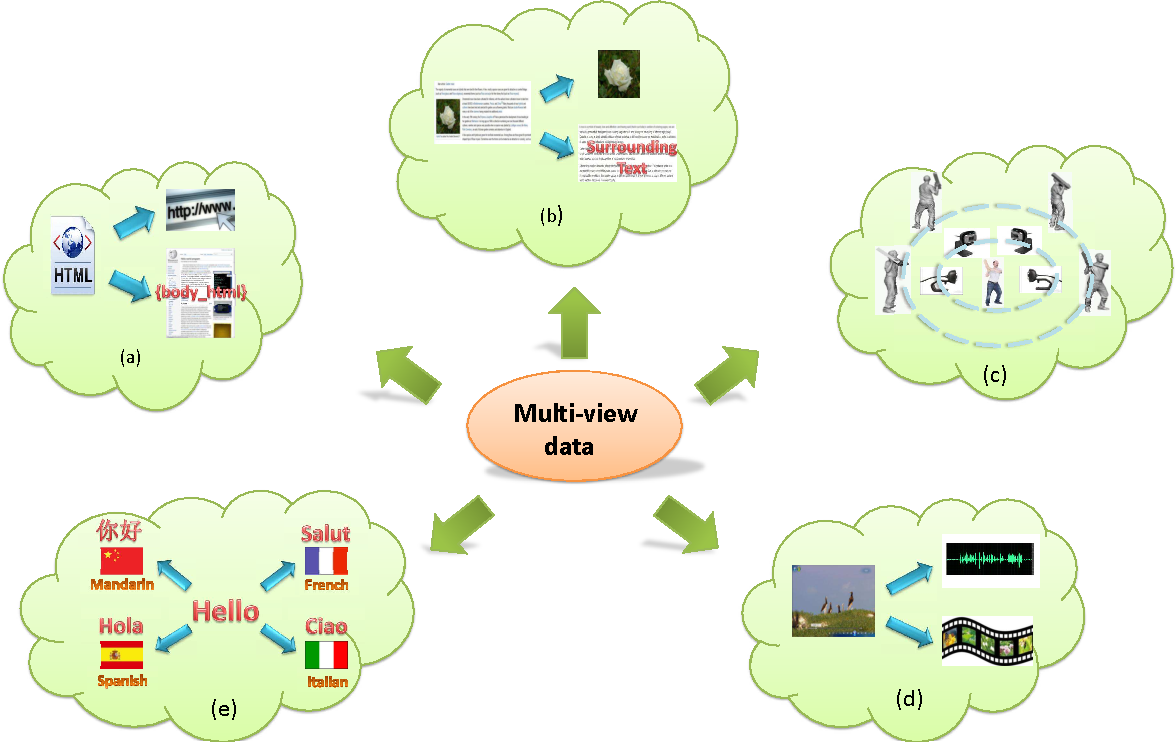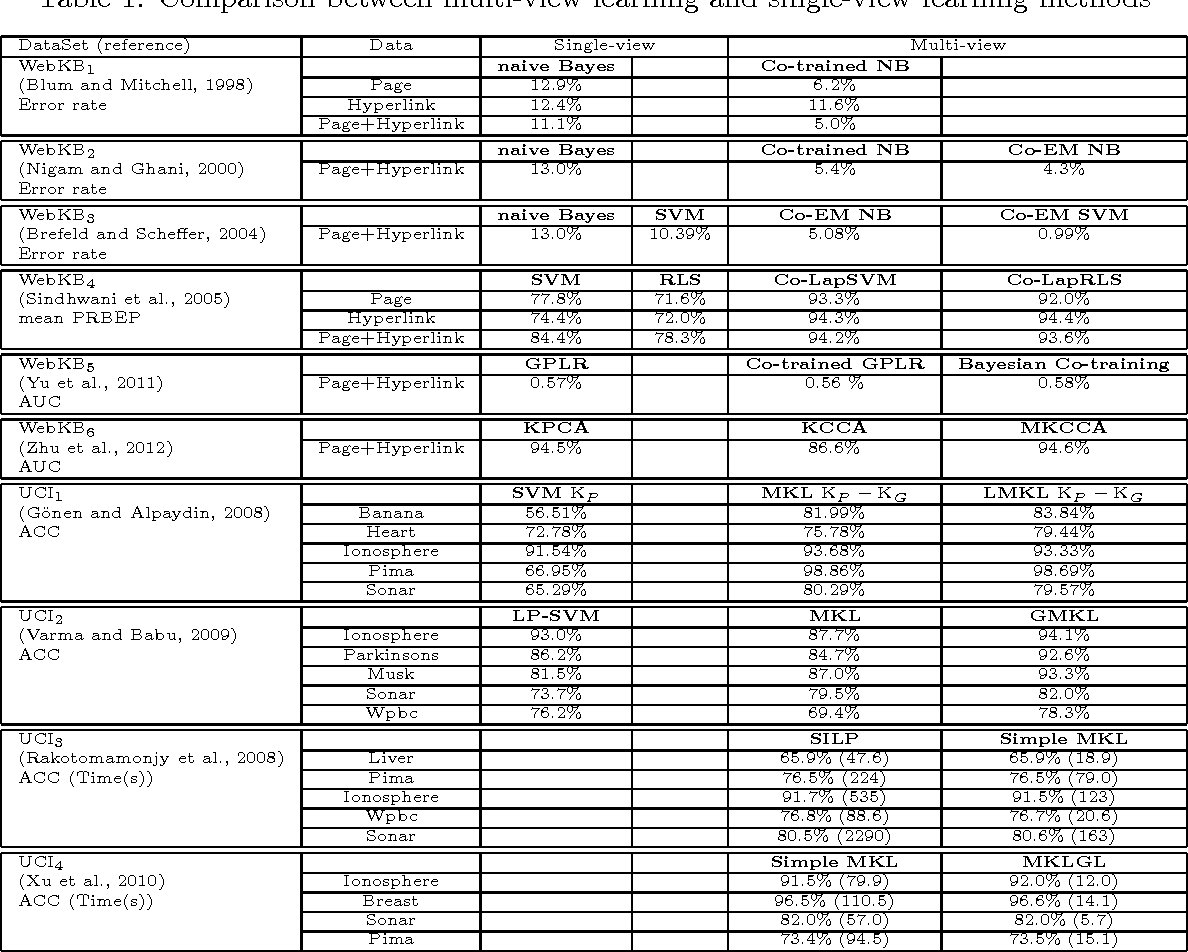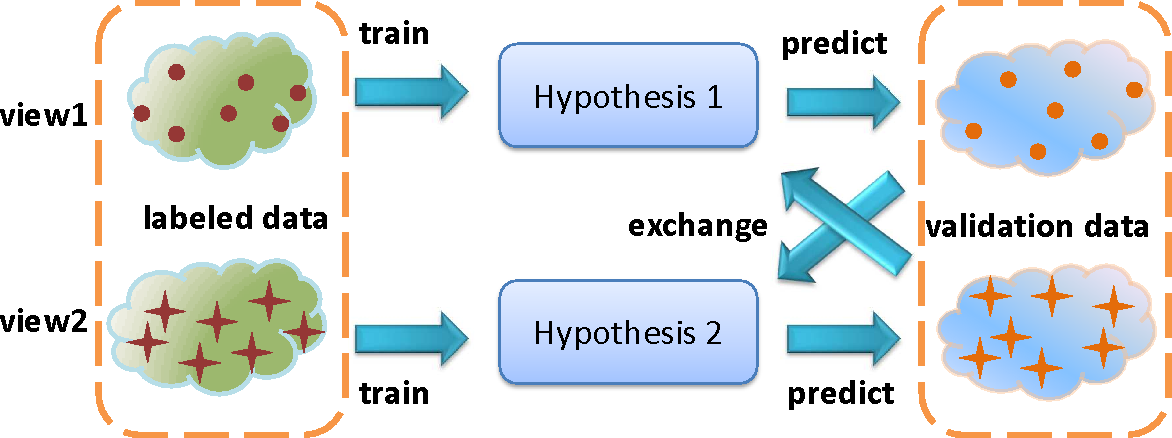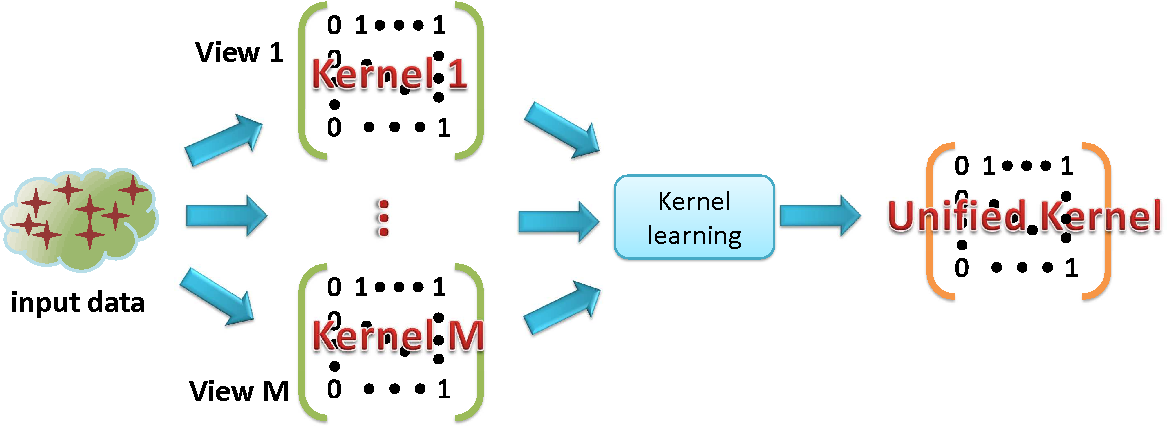In recent years, a great many methods of learning from multi-view data by considering the diversity of different views have been proposed. These views may be obtained from multiple sources or different feature subsets. In trying to organize and highlight similarities and differences between the variety of multi-view learning approaches, we review a number of representative multi-view learning algorithms in different areas and classify them into three groups: 1) co-training, 2) multiple kernel learning, and 3) subspace learning. Notably, co-training style algorithms train alternately to maximize the mutual agreement on two distinct views of the data; multiple kernel learning algorithms exploit kernels that naturally correspond to different views and combine kernels either linearly or non-linearly to improve learning performance; and subspace learning algorithms aim to obtain a latent subspace shared by multiple views by assuming that the input views are generated from this latent subspace. Though there is significant variance in the approaches to integrating multiple views to improve learning performance, they mainly exploit either the consensus principle or the complementary principle to ensure the success of multi-view learning. Since accessing multiple views is the fundament of multi-view learning, with the exception of study on learning a model from multiple views, it is also valuable to study how to construct multiple views and how to evaluate these views. Overall, by exploring the consistency and complementary properties of different views, multi-view learning is rendered more effective, more promising, and has better generalization ability than single-view learning.

Click to Read Paper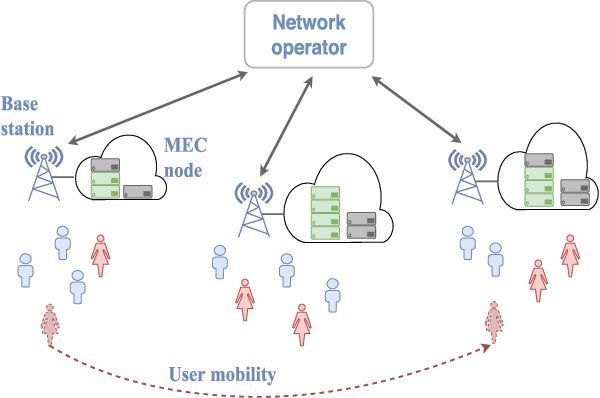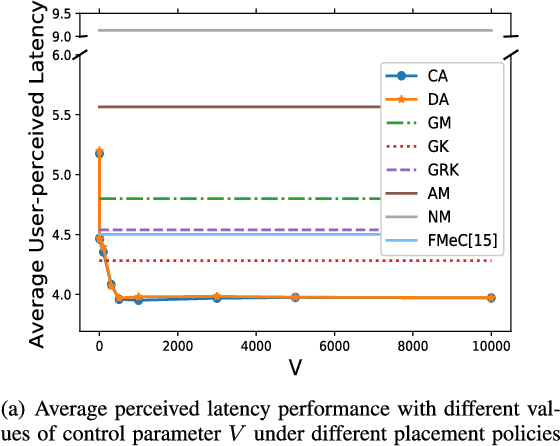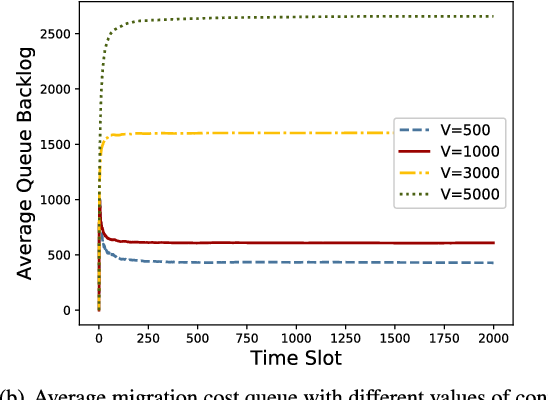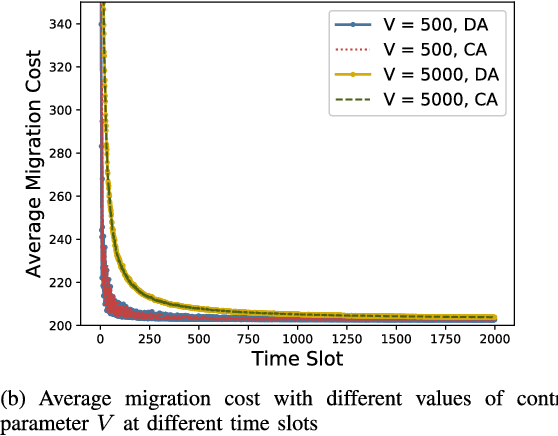Mobile edge computing is a new computing paradigm, which pushes cloud computing capabilities away from the centralized cloud to the network edge. However, with the sinking of computing capabilities, the new challenge incurred by user mobility arises: since end-users typically move erratically, the services should be dynamically migrated among multiple edges to maintain the service performance, i.e., user-perceived latency. Tackling this problem is non-trivial since frequent service migration would greatly increase the operational cost. To address this challenge in terms of the performance-cost trade-off, in this paper we study the mobile edge service performance optimization problem under long-term cost budget constraint. To address user mobility which is typically unpredictable, we apply Lyapunov optimization to decompose the long-term optimization problem into a series of real-time optimization problems which do not require a priori knowledge such as user mobility. As the decomposed problem is NP-hard, we first design an approximation algorithm based on Markov approximation to seek a near-optimal solution. To make our solution scalable and amenable to future 5G application scenario with large-scale user devices, we further propose a distributed approximation scheme with greatly reduced time complexity, based on the technique of best response update. Rigorous theoretical analysis and extensive evaluations demonstrate the efficacy of the proposed centralized and distributed schemes.

* The paper is accepted by IEEE Journal on Selected Areas in Communications, Aug. 2018
Click to Read Paper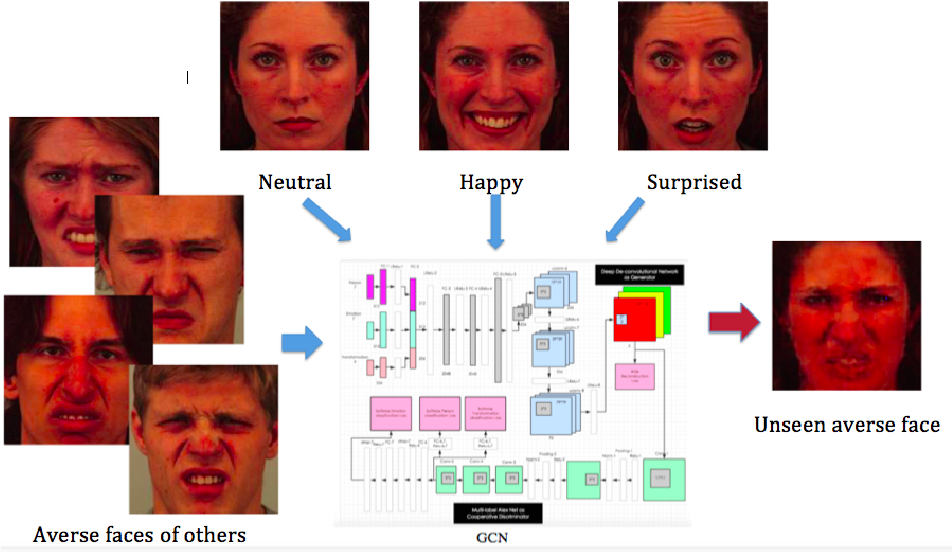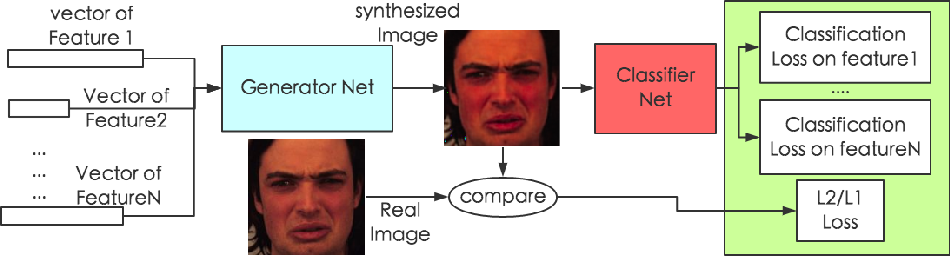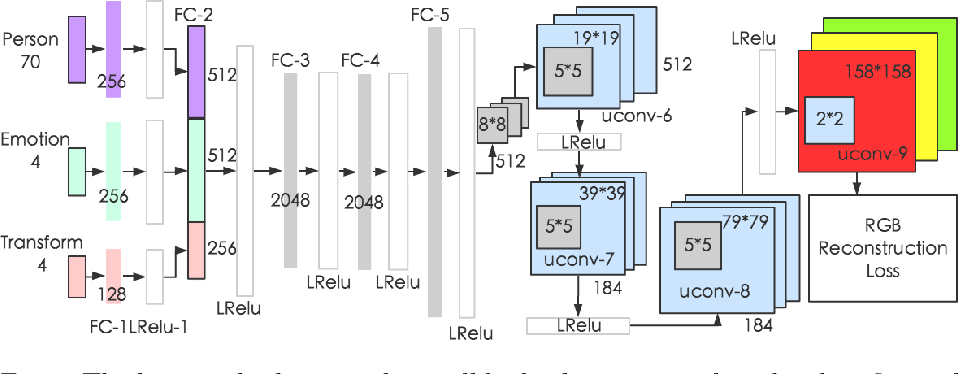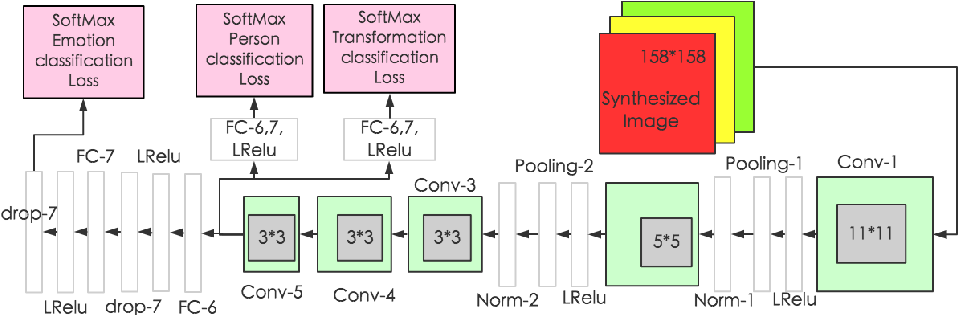How to build a good model for image generation given an abstract concept is a fundamental problem in computer vision. In this paper, we explore a generative model for the task of generating unseen images with desired features. We propose the Generative Cooperative Net (GCN) for image generation. The idea is similar to generative adversarial networks except that the generators and discriminators are trained to work accordingly. Our experiments on hand-written digit generation and facial expression generation show that GCN's two cooperative counterparts (the generator and the classifier) can work together nicely and achieve promising results. We also discovered a usage of such generative model as an data-augmentation tool. Our experiment of applying this method on a recognition task shows that it is very effective comparing to other existing methods. It is easy to set up and could help generate a very large synthesized dataset.

* 12 pages, 8 figures
Click to Read Paper
The $k$-dimensional coding schemes refer to a collection of methods that attempt to represent data using a set of representative $k$-dimensional vectors, and include non-negative matrix factorization, dictionary learning, sparse coding, $k$-means clustering and vector quantization as special cases. Previous generalization bounds for the reconstruction error of the $k$-dimensional coding schemes are mainly dimensionality independent. A major advantage of these bounds is that they can be used to analyze the generalization error when data is mapped into an infinite- or high-dimensional feature space. However, many applications use finite-dimensional data features. Can we obtain dimensionality-dependent generalization bounds for $k$-dimensional coding schemes that are tighter than dimensionality-independent bounds when data is in a finite-dimensional feature space? The answer is positive. In this paper, we address this problem and derive a dimensionality-dependent generalization bound for $k$-dimensional coding schemes by bounding the covering number of the loss function class induced by the reconstruction error. The bound is of order $\mathcal{O}\left(\left(mk\ln(mkn)/n\right)^{\lambda_n}\right)$, where $m$ is the dimension of features, $k$ is the number of the columns in the linear implementation of coding schemes, $n$ is the size of sample, $\lambda_n>0.5$ when $n$ is finite and $\lambda_n=0.5$ when $n$ is infinite. We show that our bound can be tighter than previous results, because it avoids inducing the worst-case upper bound on $k$ of the loss function and converges faster. The proposed generalization bound is also applied to some specific coding schemes to demonstrate that the dimensionality-dependent bound is an indispensable complement to these dimensionality-independent generalization bounds.

Click to Read Paper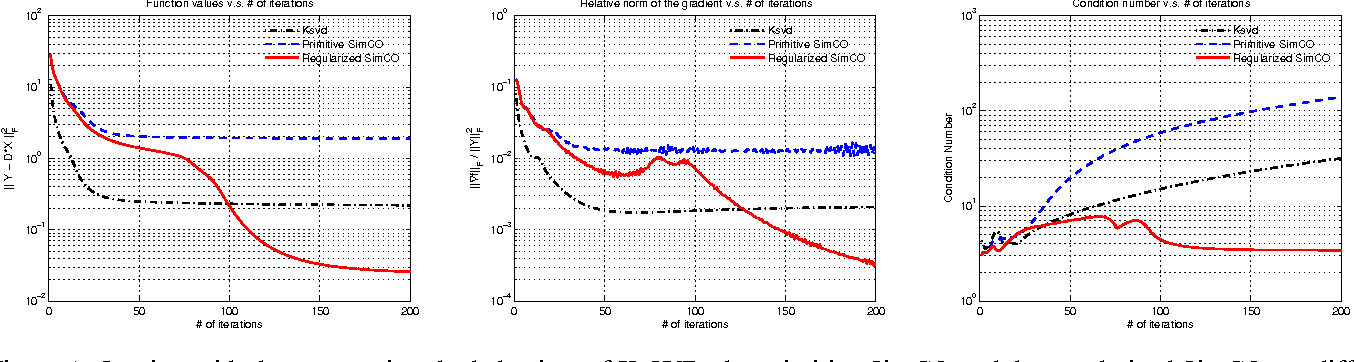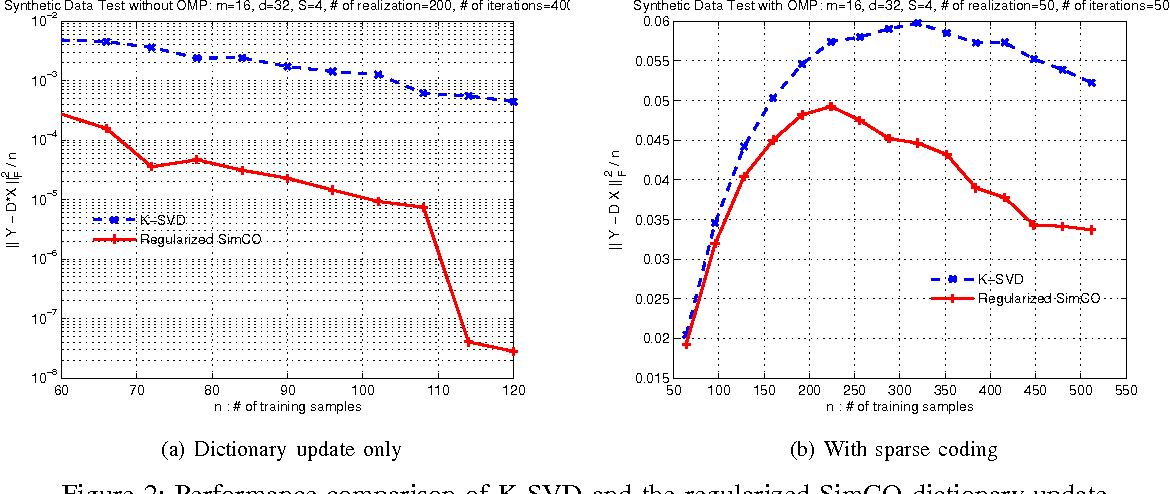We consider the data-driven dictionary learning problem. The goal is to seek an over-complete dictionary from which every training signal can be best approximated by a linear combination of only a few codewords. This task is often achieved by iteratively executing two operations: sparse coding and dictionary update. In the literature, there are two benchmark mechanisms to update a dictionary. The first approach, such as the MOD algorithm, is characterized by searching for the optimal codewords while fixing the sparse coefficients. In the second approach, represented by the K-SVD method, one codeword and the related sparse coefficients are simultaneously updated while all other codewords and coefficients remain unchanged. We propose a novel framework that generalizes the aforementioned two methods. The unique feature of our approach is that one can update an arbitrary set of codewords and the corresponding sparse coefficients simultaneously: when sparse coefficients are fixed, the underlying optimization problem is similar to that in the MOD algorithm; when only one codeword is selected for update, it can be proved that the proposed algorithm is equivalent to the K-SVD method; and more importantly, our method allows us to update all codewords and all sparse coefficients simultaneously, hence the term simultaneous codeword optimization (SimCO). Under the proposed framework, we design two algorithms, namely, primitive and regularized SimCO. We implement these two algorithms based on a simple gradient descent mechanism. Simulations are provided to demonstrate the performance of the proposed algorithms, as compared with two baseline algorithms MOD and K-SVD. Results show that regularized SimCO is particularly appealing in terms of both learning performance and running speed.

* 13 pages
Click to Read Paper
Maximum-likelihood estimation (MLE) is widely used in sequence to sequence tasks for model training. It uniformly treats the generation/prediction of each target token as multi-class classification, and yields non-smooth prediction probabilities: in a target sequence, some tokens are predicted with small probabilities while other tokens are with large probabilities. According to our empirical study, we find that the non-smoothness of the probabilities results in low quality of generated sequences. In this paper, we propose a sentence-wise regularization method which aims to output smooth prediction probabilities for all the tokens in the target sequence. Our proposed method can automatically adjust the weights and gradients of each token in one sentence to ensure the predictions in a sequence uniformly well. Experiments on three neural machine translation tasks and one text summarization task show that our method outperforms conventional MLE loss on all these tasks and achieves promising BLEU scores on WMT14 English-German and WMT17 Chinese-English translation task.

* AAAI 2019
Click to Read Paper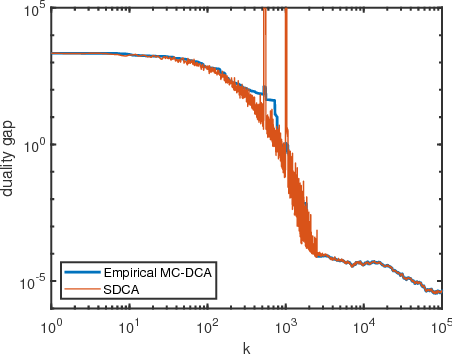The method of block coordinate gradient descent (BCD) has been a powerful method for large-scale optimization. This paper considers the BCD method that successively updates a series of blocks selected according to a Markov chain. This kind of block selection is neither i.i.d. random nor cyclic. On the other hand, it is a natural choice for some applications in distributed optimization and Markov decision process, where i.i.d. random and cyclic selections are either infeasible or very expensive. By applying mixing-time properties of a Markov chain, we prove convergence of Markov chain BCD for minimizing Lipschitz differentiable functions, which can be nonconvex. When the functions are convex and strongly convex, we establish both sublinear and linear convergence rates, respectively. We also present a method of Markov chain inertial BCD. Finally, we discuss potential applications.

Click to Read Paper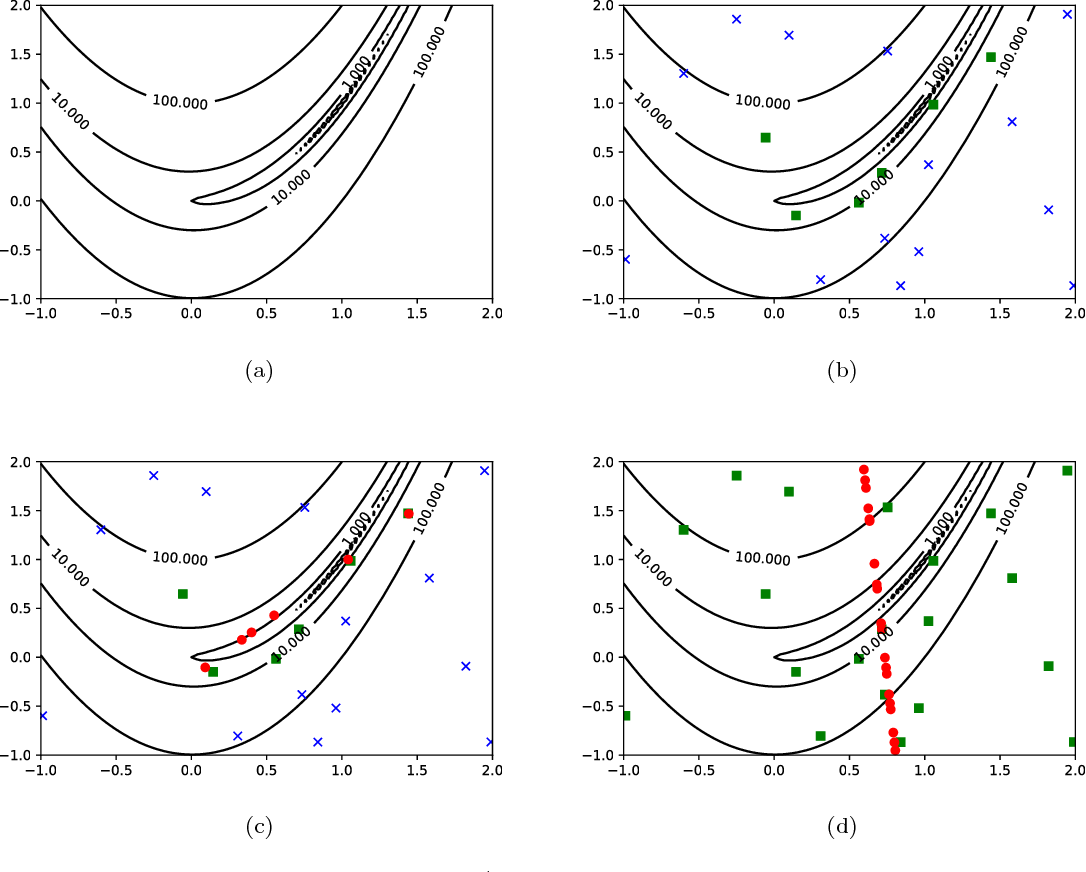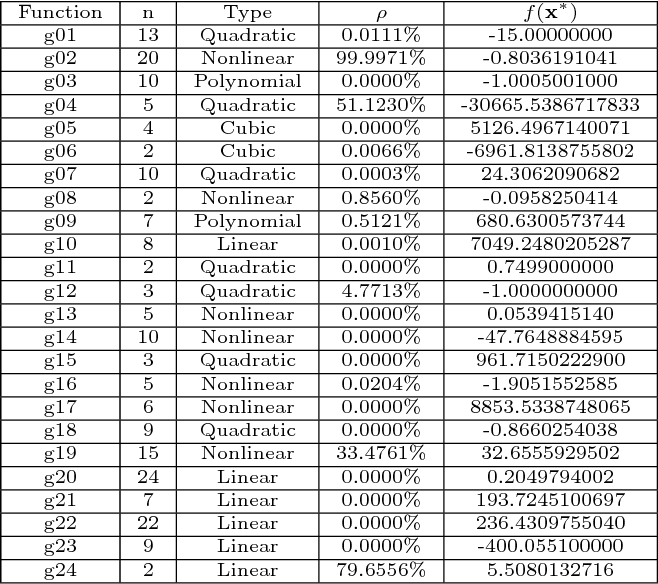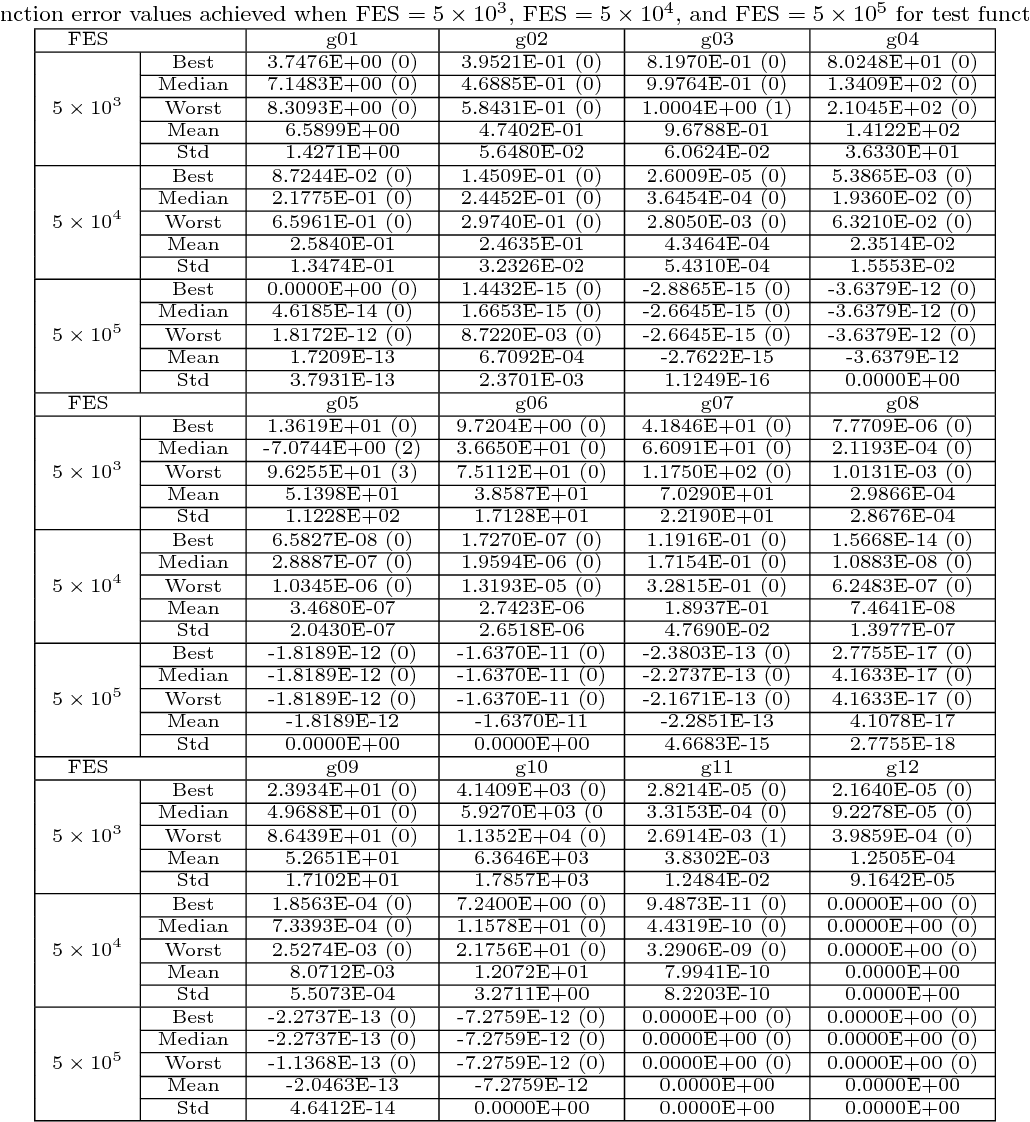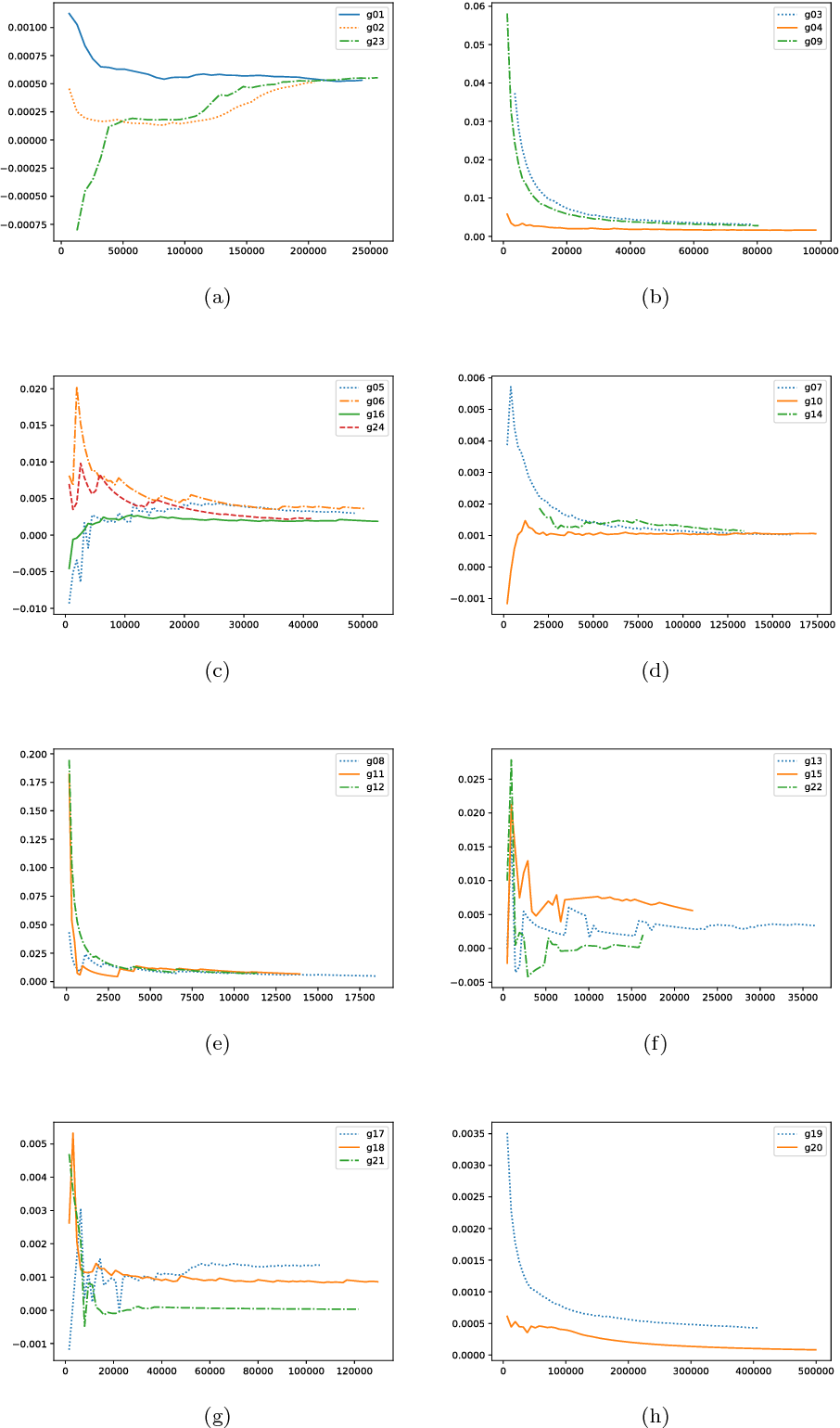Multiobjective optimization evolutionary algorithms have been successfully applied to solving constrained optimization problems. This paper proposes a new multiobjective optimization differential evolution algorithm for constrained optimization. Through a study of fitness landscapes using principle component analysis, we discover a statistic method of identifying the valley direction in a valley landscape. Based on this discovery, a new search operator called PCA-projection is constructed which projects an individual to a position along the valley direction. Then multiobjective optimization differential evolution using this projection operator is designed for constrained optimization. A comparative experiment has been implemented between the proposed algorithm and a state-of-the-art multiobjective differential evolution algorithm on a standard set of 24 benchmarks. Experimental results show that the new algorithm makes a significant improvement in terms of solution accuracy. The proposed algorithm is also competitive with ten evolutionary algorithms participated in an IEEE CEC 2006 competition and is ranked third in terms of the final rank.

Click to Read Paper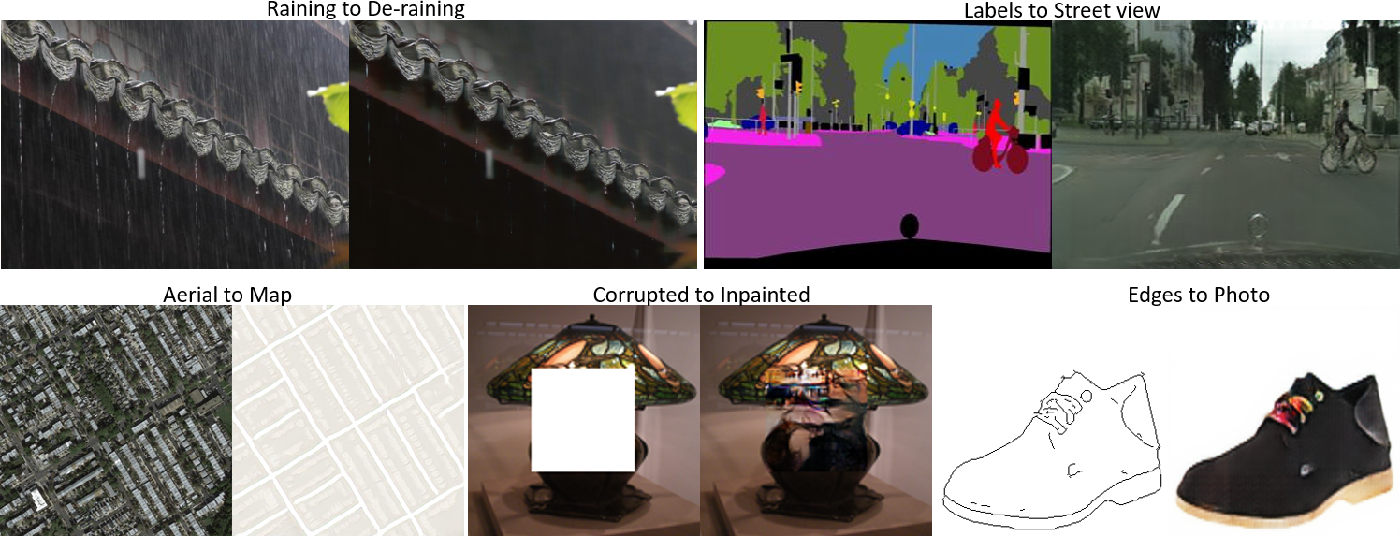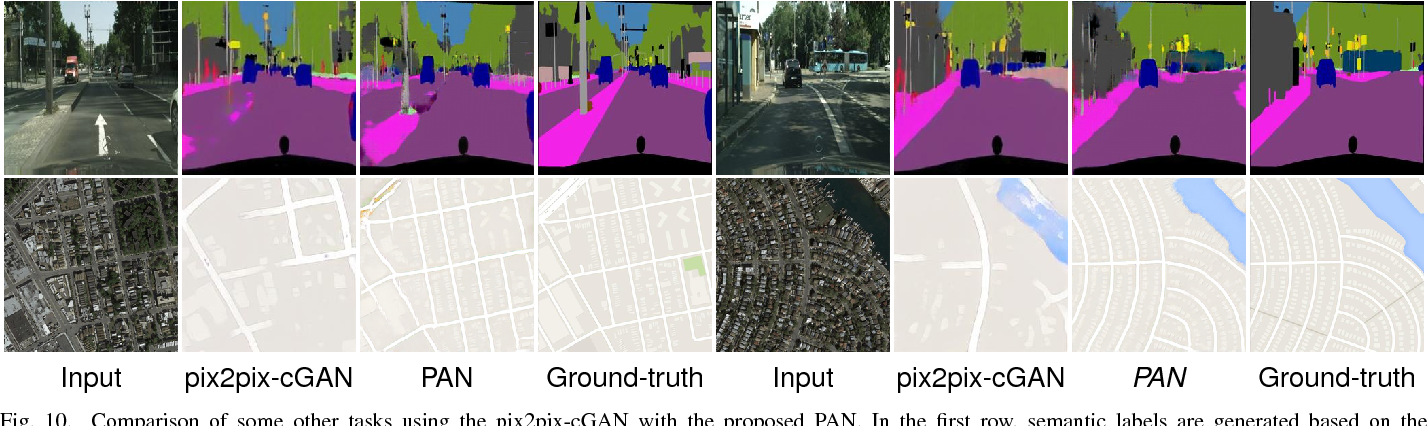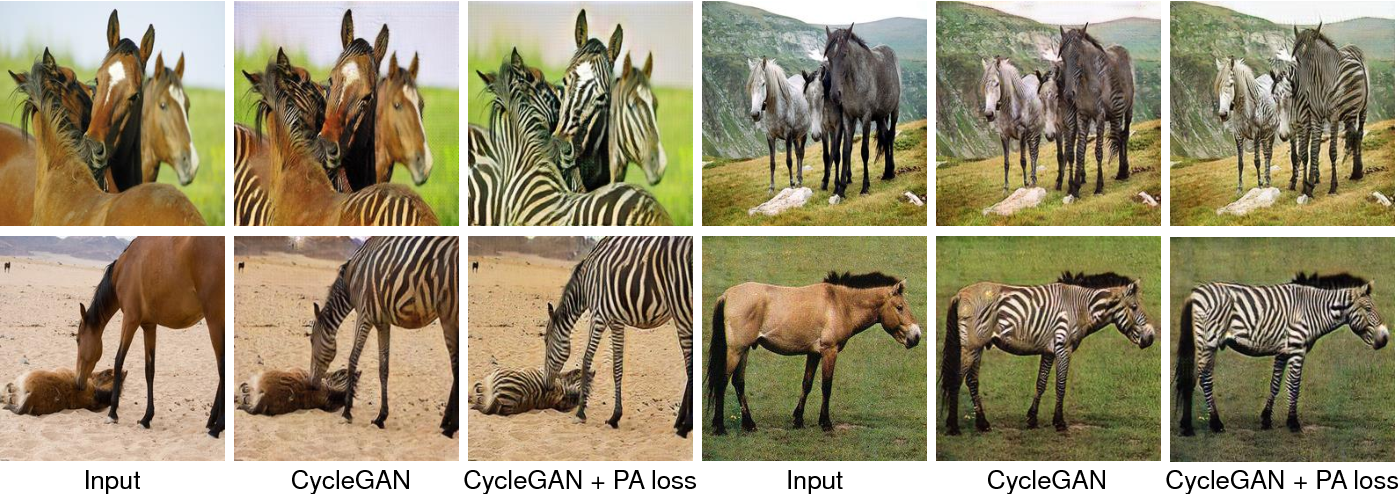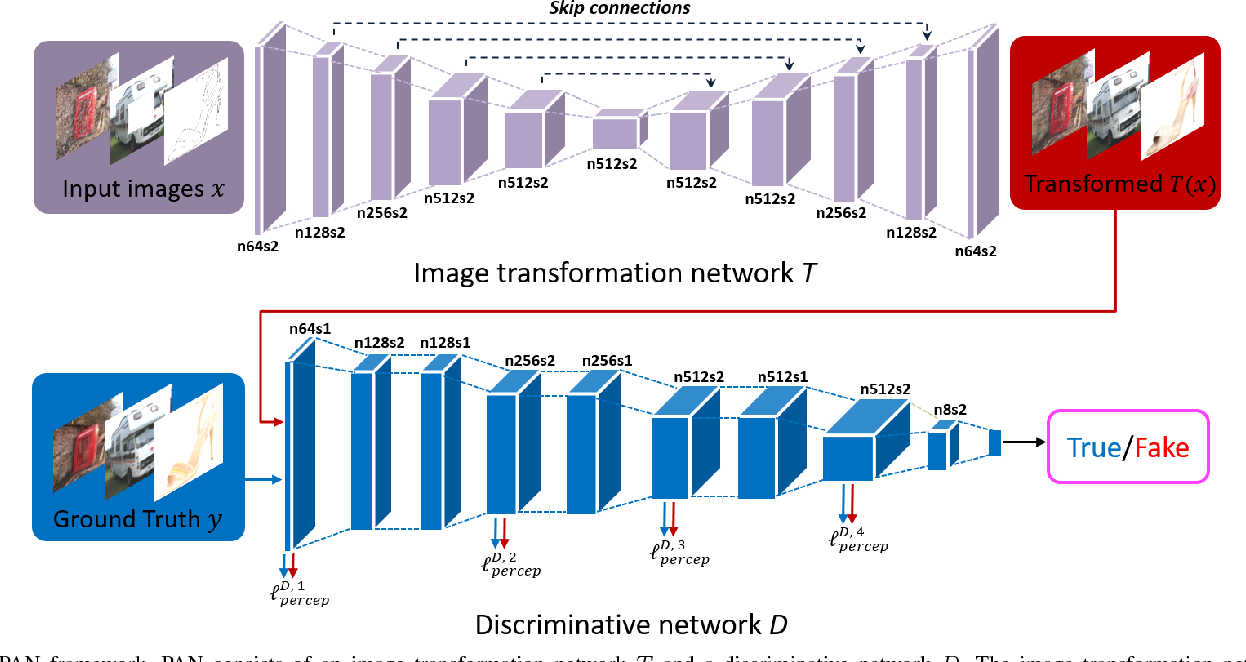In this paper, we propose a principled Perceptual Adversarial Networks (PAN) for image-to-image transformation tasks. Unlike existing application-specific algorithms, PAN provides a generic framework of learning mapping relationship between paired images (Fig. 1), such as mapping a rainy image to its de-rained counterpart, object edges to its photo, semantic labels to a scenes image, etc. The proposed PAN consists of two feed-forward convolutional neural networks (CNNs), the image transformation network T and the discriminative network D. Through combining the generative adversarial loss and the proposed perceptual adversarial loss, these two networks can be trained alternately to solve image-to-image transformation tasks. Among them, the hidden layers and output of the discriminative network D are upgraded to continually and automatically discover the discrepancy between the transformed image and the corresponding ground-truth. Simultaneously, the image transformation network T is trained to minimize the discrepancy explored by the discriminative network D. Through the adversarial training process, the image transformation network T will continually narrow the gap between transformed images and ground-truth images. Experiments evaluated on several image-to-image transformation tasks (e.g., image de-raining, image inpainting, etc.) show that the proposed PAN outperforms many related state-of-the-art methods.

* 20 pages, 9 figures
Click to Read Paper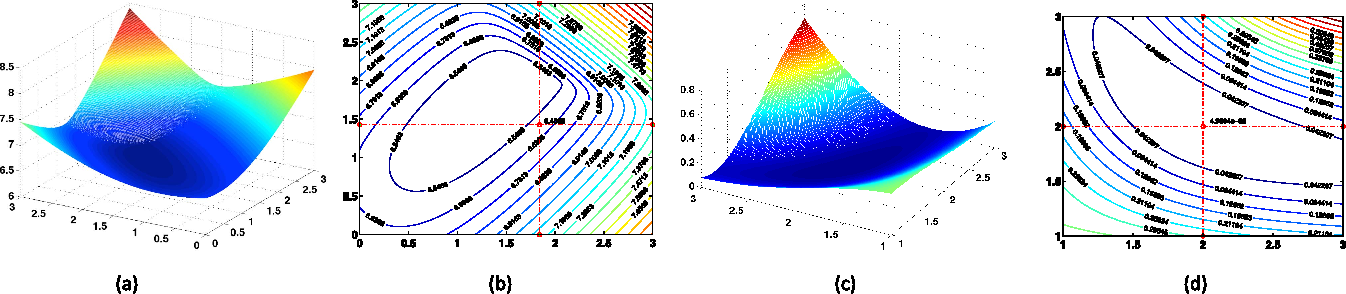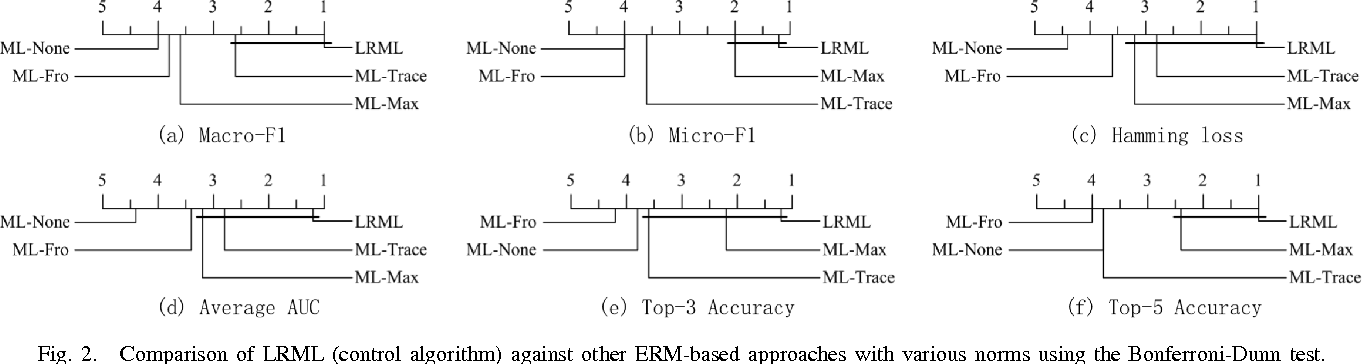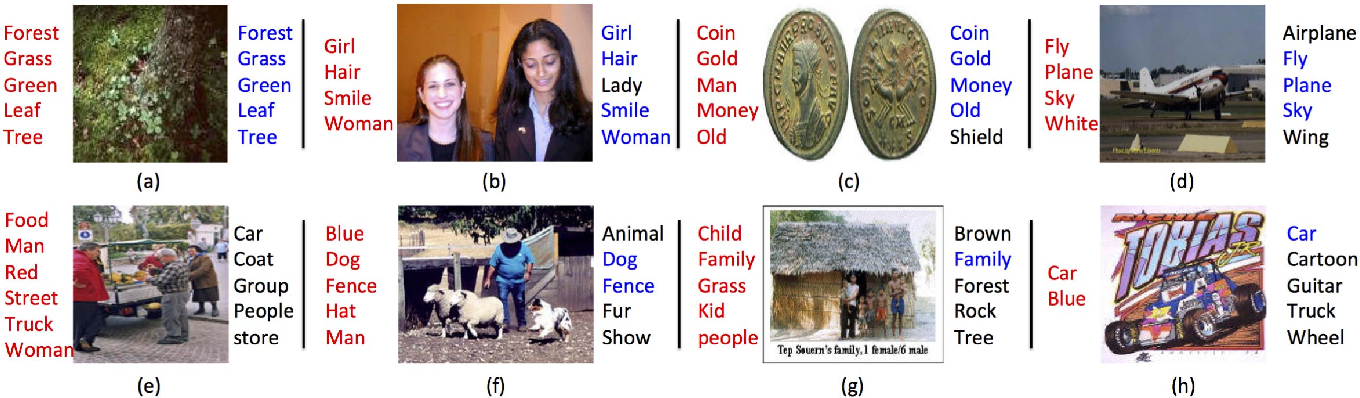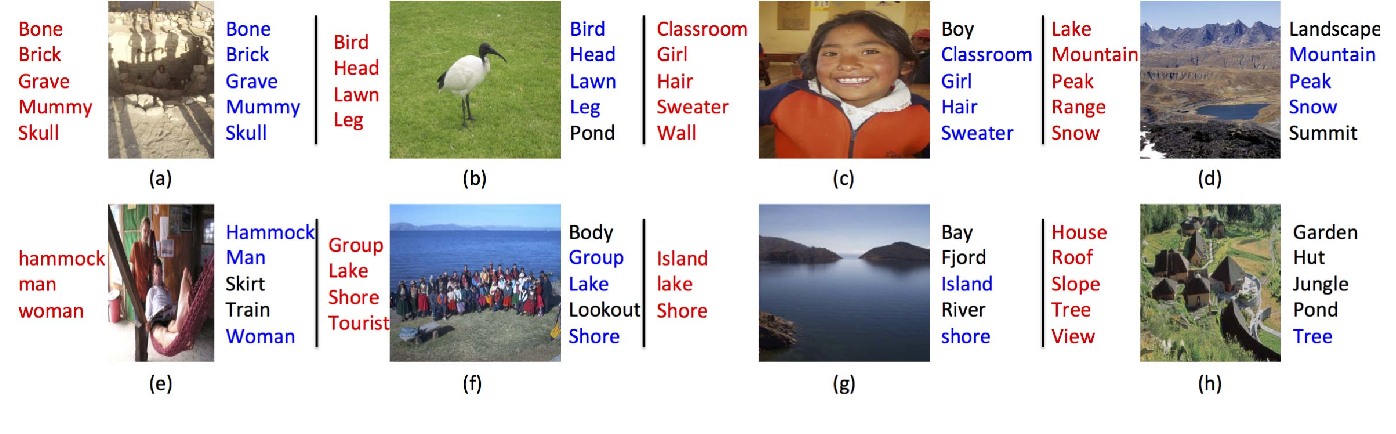We analyze the local Rademacher complexity of empirical risk minimization (ERM)-based multi-label learning algorithms, and in doing so propose a new algorithm for multi-label learning. Rather than using the trace norm to regularize the multi-label predictor, we instead minimize the tail sum of the singular values of the predictor in multi-label learning. Benefiting from the use of the local Rademacher complexity, our algorithm, therefore, has a sharper generalization error bound and a faster convergence rate. Compared to methods that minimize over all singular values, concentrating on the tail singular values results in better recovery of the low-rank structure of the multi-label predictor, which plays an import role in exploiting label correlations. We propose a new conditional singular value thresholding algorithm to solve the resulting objective function. Empirical studies on real-world datasets validate our theoretical results and demonstrate the effectiveness of the proposed algorithm.

Click to Read Paper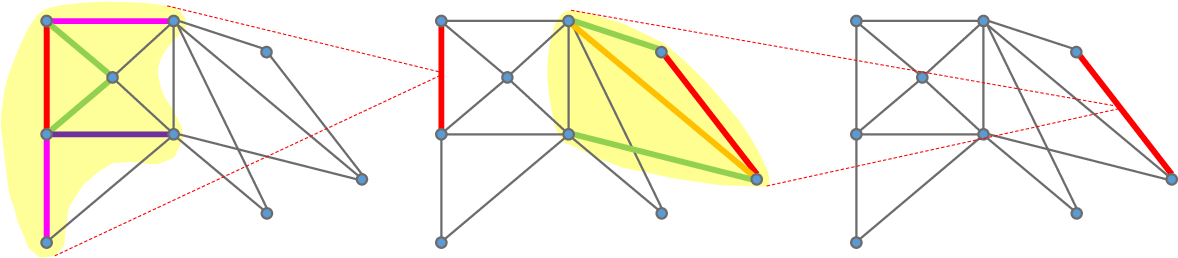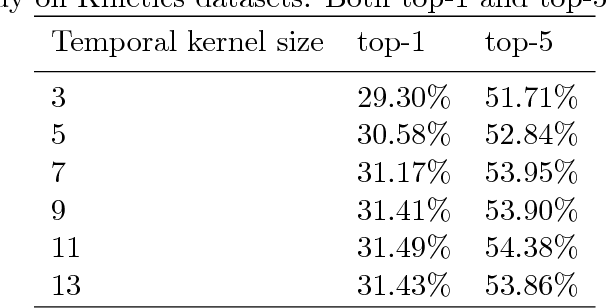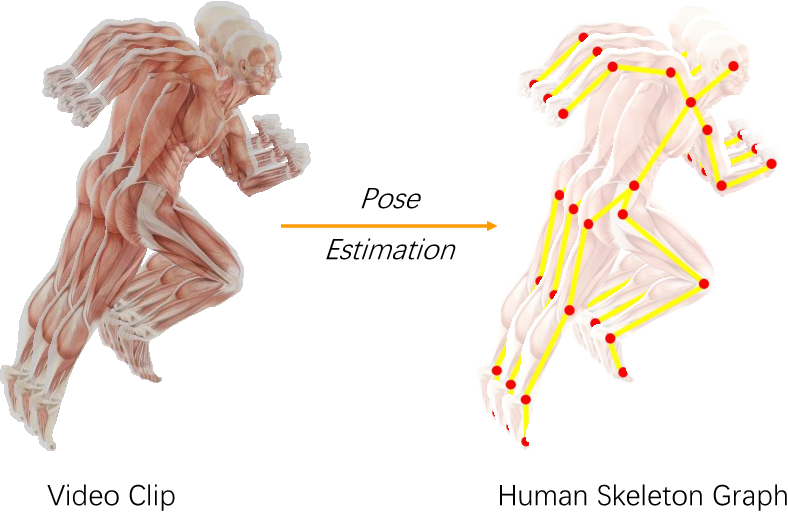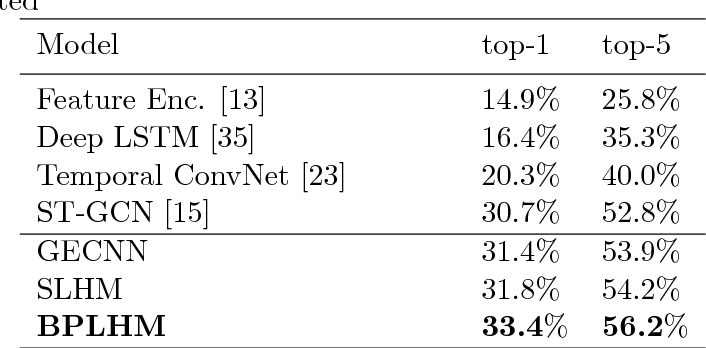This paper investigates body bones from skeleton data for skeleton based action recognition. Body joints, as the direct result of mature pose estimation technologies, are always the key concerns of traditional action recognition methods. However, instead of joints, we humans naturally identify how the human body moves according to shapes, lengths and places of bones, which are more obvious and stable for observation. Hence given graphs generated from skeleton data, we propose to develop convolutions over graph edges that correspond to bones in human skeleton. We describe an edge by integrating its spatial neighboring edges to explore the cooperation between different bones, as well as its temporal neighboring edges to address the consistency of movements in an action. A graph edge convolutional neural network is then designed for skeleton based action recognition. Considering the complementarity between graph node convolution and graph edge convolution, we additionally construct two hybrid neural networks to combine graph node convolutional neural network and graph edge convolutional neural network using shared intermediate layers. Experimental results on Kinetics and NTU-RGB+D datasets demonstrate that our graph edge convolution is effective to capture characteristic of actions and our graph edge convolutional neural network significantly outperforms existing state-of-art skeleton based action recognition methods. Additionally, more performance improvements can be achieved by the hybrid networks.

Click to Read Paper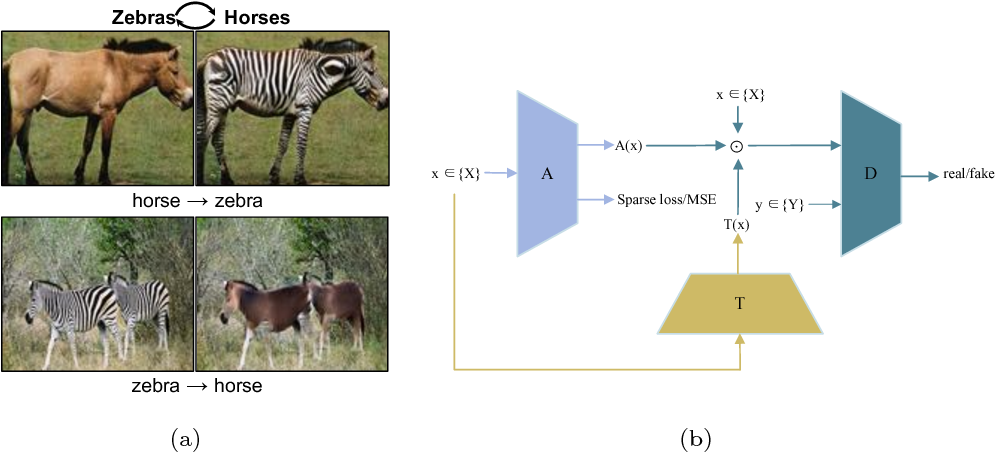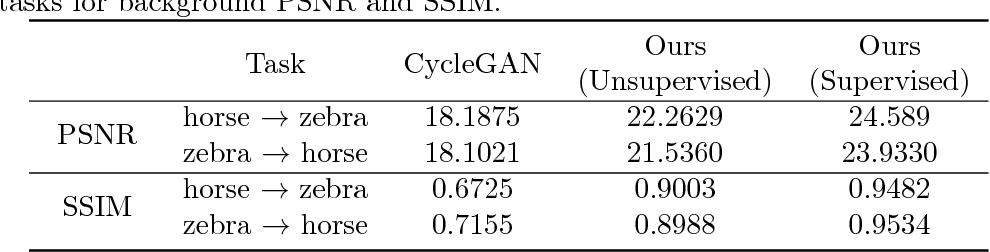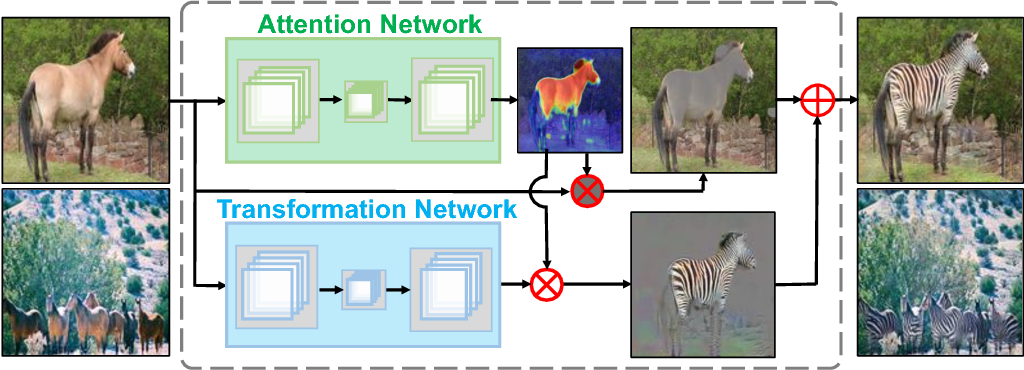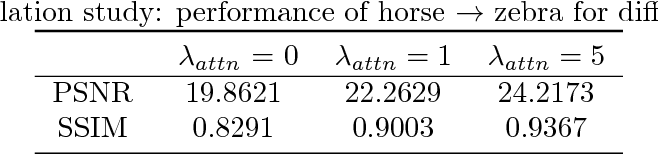This paper studies the object transfiguration problem in wild images. The generative network in classical GANs for object transfiguration often undertakes a dual responsibility: to detect the objects of interests and to convert the object from source domain to target domain. In contrast, we decompose the generative network into two separat networks, each of which is only dedicated to one particular sub-task. The attention network predicts spatial attention maps of images, and the transformation network focuses on translating objects. Attention maps produced by attention network are encouraged to be sparse, so that major attention can be paid to objects of interests. No matter before or after object transfiguration, attention maps should remain constant. In addition, learning attention network can receive more instructions, given the available segmentation annotations of images. Experimental results demonstrate the necessity of investigating attention in object transfiguration, and that the proposed algorithm can learn accurate attention to improve quality of generated images.

Click to Read Paper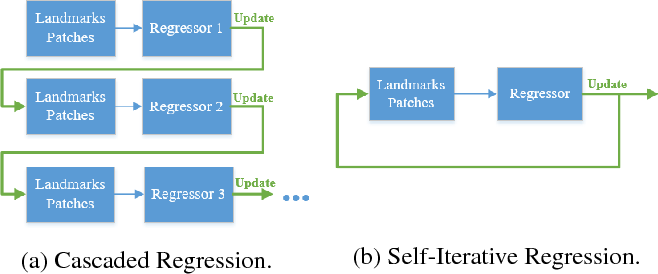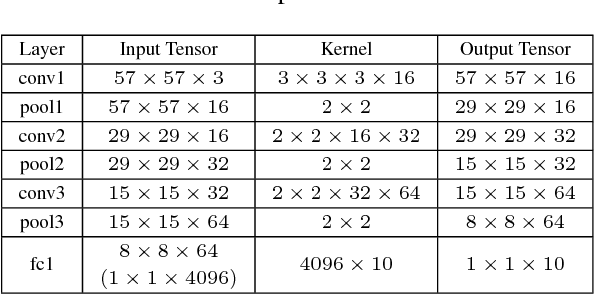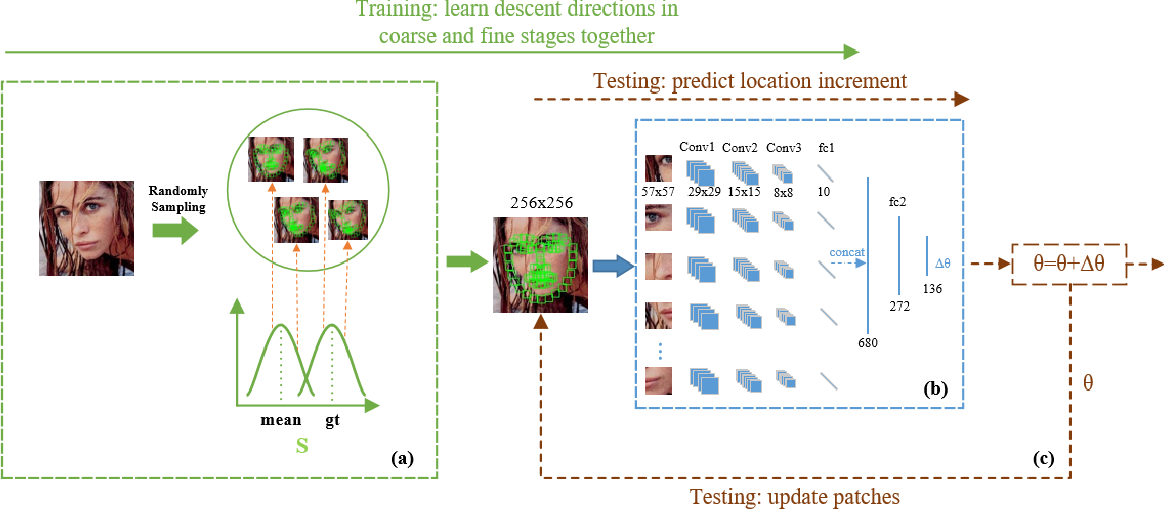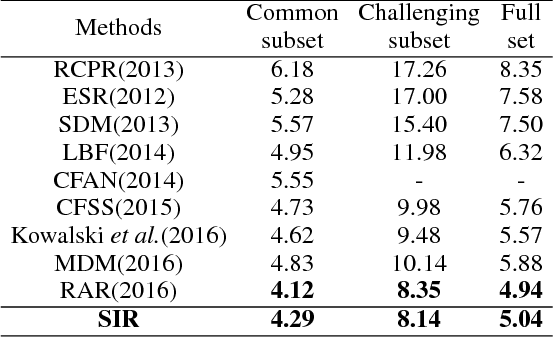Cascaded Regression (CR) based methods have been proposed to solve facial landmarks detection problem, which learn a series of descent directions by multiple cascaded regressors separately trained in coarse and fine stages. They outperform the traditional gradient descent based methods in both accuracy and running speed. However, cascaded regression is not robust enough because each regressor's training data comes from the output of previous regressor. Moreover, training multiple regressors requires lots of computing resources, especially for deep learning based methods. In this paper, we develop a Self-Iterative Regression (SIR) framework to improve the model efficiency. Only one self-iterative regressor is trained to learn the descent directions for samples from coarse stages to fine stages, and parameters are iteratively updated by the same regressor. Specifically, we proposed Landmarks-Attention Network (LAN) as our regressor, which concurrently learns features around each landmark and obtains the holistic location increment. By doing so, not only the rest of regressors are removed to simplify the training process, but the number of model parameters is significantly decreased. The experiments demonstrate that with only 3.72M model parameters, our proposed method achieves the state-of-the-art performance.

* Accepted in AAAI 2018
Click to Read Paper